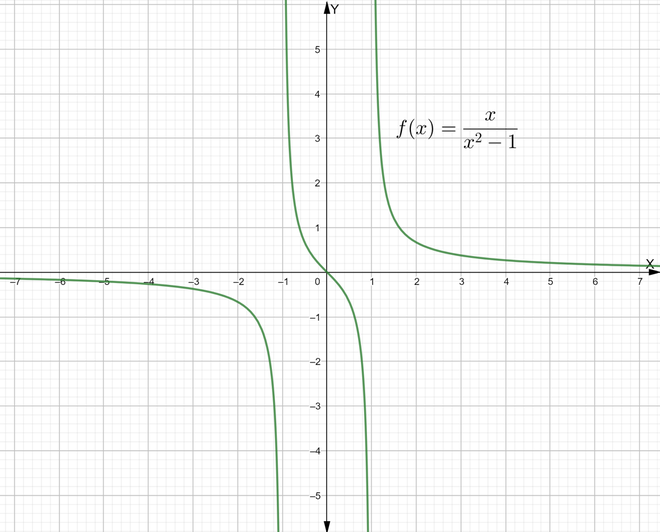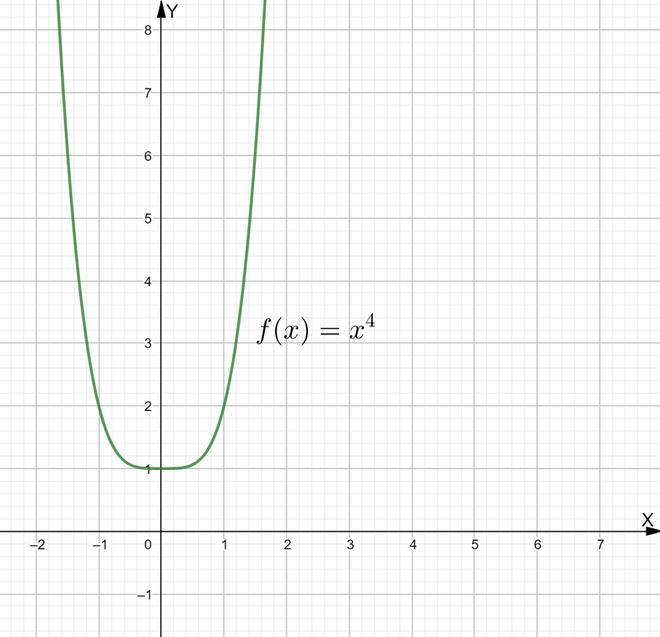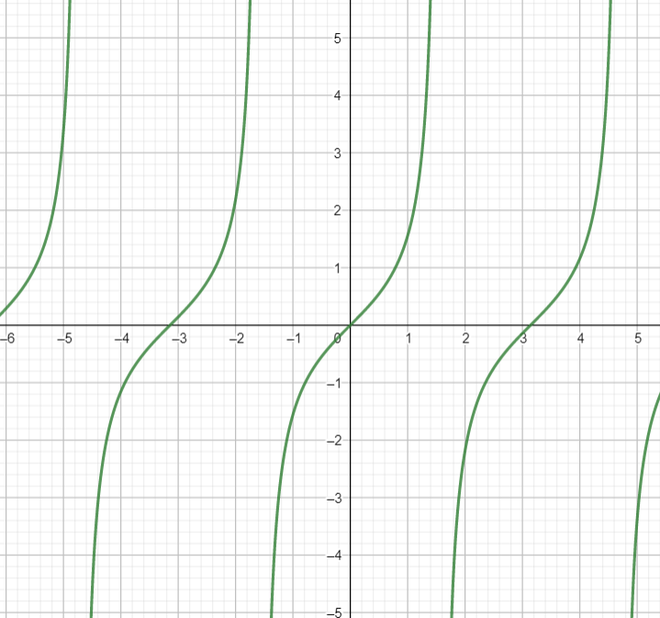# Fourier Series Formula

• Last Updated : 13 May, 2022

A Fourier series is a sum of sine and cosine waves that represents a periodic function. Each wave in the sum, or harmonic, has a frequency that is an integer multiple of the periodic function’s fundamental frequency. Harmonic analysis may be used to identify the phase and amplitude of each harmonic. A Fourier series might have an unlimited number of harmonics. Summing some, but not all, of the harmonics in a function’s Fourier series, yields an approximation to that function. For example, a square wave can be approximated by utilizing the first few harmonics of the Fourier series.

### Fourier Series

As seen above, Periodic functions often appear in problems in higher mathematics. A way of dealing with these issues is to represent them in terms of basic periodic functions, which have a small range and can have a domain of all real numbers, such as sine and cosine; this leads us to the Fourier series (FS). The Fourier series is a particularly useful tool for dealing with situations involving partial differential equations.

Suppose we are given a periodic function f(x). Now as the original  function is periodic therefore,

c1​f1​(x) + … + cn​fn​(x)​

Next consider the infinite series,⇢ (1)

Consisting of 2L-periodic functions converges for all x, then the function to which it converges will be periodic of period 2L. Now as seen above we need to represent the function f(x) in such a way that the periodic function f(x) is replaced by functions like sine and cosine. For this the Fourier series is given by,Here,..

n = 1,2,3….

General form of Fourier Series

For any function f(x) with period 2L, the Fourier series is given as,.

Exponential form of Fourier Series

From the equation above,.

Now according to Euler’s formula,

e= cosθ +isinθ

Using this

f(x) =Cneinx.

Here Cn is called decomposition coefficient and is calculated as,.

### Conditions for Fourier series

Suppose a function f(x) has a period of 2π and is integrable in a period [-π, π]. Now there are two conditions.

The function f(x) with period 2π is absolutely integrable on [-π, π] so that the following Dirichlet integral of this function is finite:Next condition is that the function is a single valued, piecewise continuous (must have a finite number of jump discontinuities), and piecewise monotonic (must have a finite number of maxima and minima).

If conditions 1 and 2 are satisfied, the Fourier series for the function exists and converges to the given function. This means that the sum of the Fourier series of any given function converges back to give the same function. This is the basic definition of the Fourier series expansion. Before further understanding the concept of the Fourier Series we should first understand the concept of odd and even functions and periodic functions.

• Odd function: Suppose we are given a function y = f(x).

Now if,

f(-x) = -f(x) = -y

then the function is said to be odd.The function is odd in nature and symmetric about the origin

• Even function: Again consider a function f(x) = y.

If f(-x) = f(x) = y

Then the function is even in nature.This is an even function whose graph that is symmetric along the y-axis.

• Periodic functions: Let a function f(x) be periodic with an interval λ. Now consider an element x as a part of the domain of this function. This means that,

f(x) = f(x + λ).The graph of function tanx is an example of a periodic function.

Hence periodic functions are those functions that repeat themselves over an interval of values(λ as shown above). The smallest possible positive value of λ is called the period of this function.

### Sample problems

Question 1: Find the Fourier series expansion of the function f(x) = ex, within the limits  [– π, π].

Solution:

Using Fourier series expansion....

The Fourier series for this function is given as,.

Question 2: Find the Fourier series expansion of the function f(x) = x , within the limits  [– 1, 1].

Solution:

From Fourier series expansion. Here,.....

Om solving the integrals we get even functions and one odd function. Therefore,.

Question 3: Suppose a function f(x) = tanx find its Fourier expansion within the limits [-π, π].

Solution:Now the integral of tanx⋅sinnx and tanx⋅cosnx cannot be found.

Therefore the Fourier series for this function f(x) = tanx is undefined.

Question 4: Find the Fourier series of the function f(x) = 1 for limits  [– π, π] .

Solution:

Comparing with general Fourier series expansion we get,f(x) = π + 0 + 0

f(x) = π

Question 5: Consider a function f(x) = x2 for the limits  [– π, π]. Find its Fourier series expansion.

Solution:

Comparing with general Fourier series expansion we get,Question 6: Find Fourier series expansion of the function f(x) = 4-3x for the limits  [– 1, 1].

Solution:

Comparing with general Fourier series expansion we get,Question 7: Find the expansion of the function. For the limits  [– π, π].

Solution:

Comparing with general Fourier series expansion we get,My Personal Notes arrow_drop_up
Recommended Articles
Page :